# 数据结构课程设计

1. 需求分析: 4
1.1问题描述: 4
1.2问题要求: 4

2. 概要设计 4
2.1抽象数据类型定义 4
2.2设计思路 4
2.2.1模块调用： 4
3详细设计 6
3.1存储结构设计 6
3.1.1顺序查找的基本思想 6
3.1.2二分法查找（折半查找）的基本思想 7
3.1.3斐波那契查找的前提是待查找的查找表必须顺序存储并且有序 9
3.1.4二叉排序树简介 10
3.2二叉排序树相关操作 11
3.3功能模块设计 20
4.运行与测试 27
5.总结 32
6.附录 32

3. 需求分析:
1.1问题描述:
设计一个实现①顺序查找②二分查找（折半查找）、④二叉排序树、⑤平衡二叉树、③哈希查找算法的程序，并具有人机交互界面。
基本要求：
（1）设计一个菜单将实现的查找算法的名字显示出来，并提示用户对查找算法进行选择；
（2）分别实现顺序查找、二分查找（折半查找）、二叉排序树、哈希查找；
（3）哈希函数采用除留余数发，解决冲突的方法大家任选择一种；
（4）二叉排序树必须实现构建、查找、插入、删除四个基本操作；
（5）输出各种排序的结果并进行比较。
要求：如何用多种数据结构来求解问题。同时要求实现对应数据结构的所有基本操作。
1.2问题要求:
(1) 建立顺序表，设置关键字来实现顺序查找。
(2) 运用上述建立的顺序表，来实现二分查找。
(3) 建立链表结构，综合运用队列的相关知识，实现二叉树的排序。
(4) 运用除留余数法来分配空间，线性探测法来解决冲突实现哈希查找
(5) 在主函数中调用用户自定义函数，输出相应结果
(6) 本程序是对几种查找算法的综合比较

4. 概要设计
2.1抽象数据类型定义
查询数据类型定义：
数据对象：D={aij|aij属于{1，2，3…},i,j>0}
数据关系：R={<ai-1,ai>|ai-1,ai∈D，i=2,…,n}
2.2设计思路
2.2.1模块调用：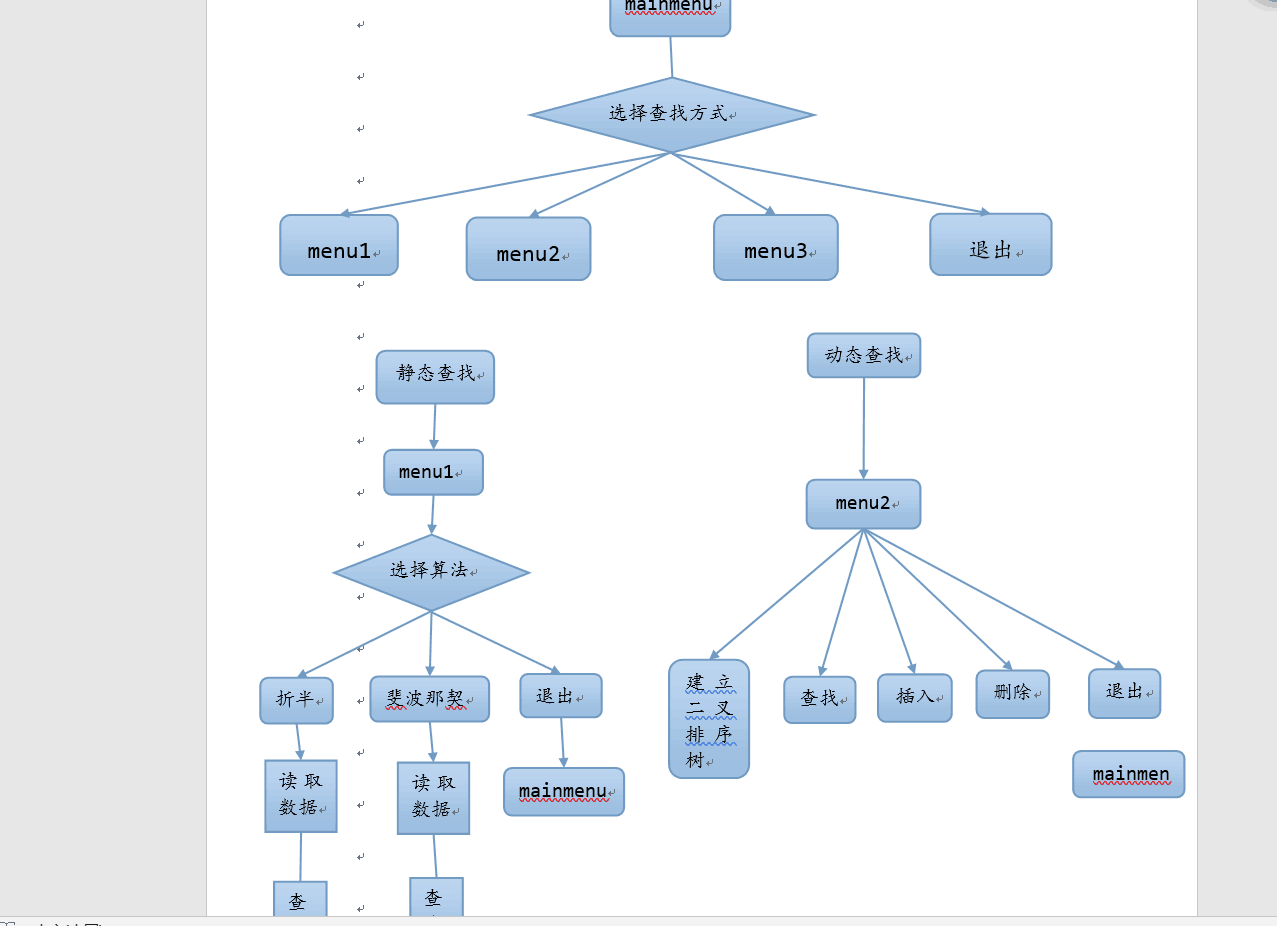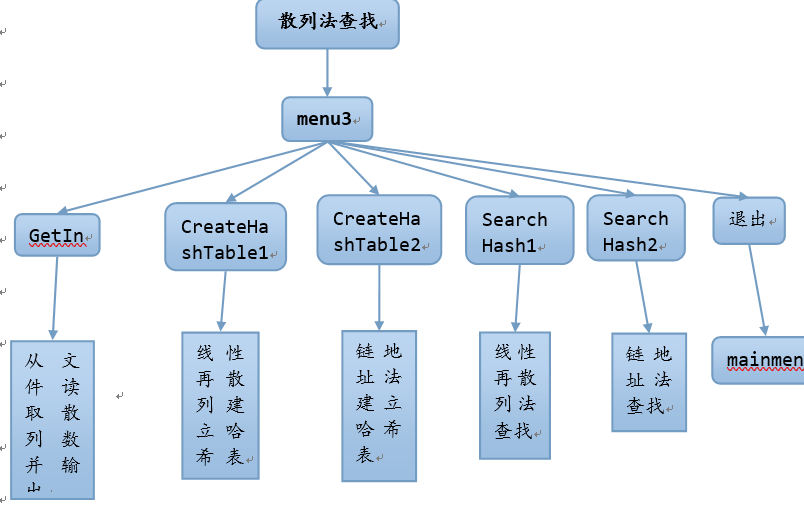3详细设计
3.1存储结构设计
3.1.1顺序查找的基本思想
从表的一端开始，顺序扫描表，依次将扫描到的结点关键字和给定值（假定为a）相比较，若当前结点关键字与a相等，则查找成功；若扫描结束后，仍未找到关键字等于a的结点，则查找失败。

1 2 3 4 5 6 7 8 9 10 11 12 13 14 15 16

int Find(int[] ary, int target)
{
for (int i = 0; i < ary.Length; i++)
{
if (ary[i] == target)
{
//找到了就返回找到的位置
return i;
}
}
//没找到就返回-1，表示没找到
return -1;
}
3.1.2二分法查找（折半查找）的基本思想

（1）确定该区间的中点位置：mid=（low+high）/2
min代表区间中间的结点的位置，low代表区间最左结点位置，high代表区间最右结点位置
（2）将待查a值与结点mid的关键字（下面用R[mid].key）比较，若相等，则查找成功，否则确定新的查找区间：

（3）下一次查找针对新的查找区间，重复步骤（1）和（2）
（4）在查找过程中，low逐步增加，high逐步减少，如果high<low，则查找失败。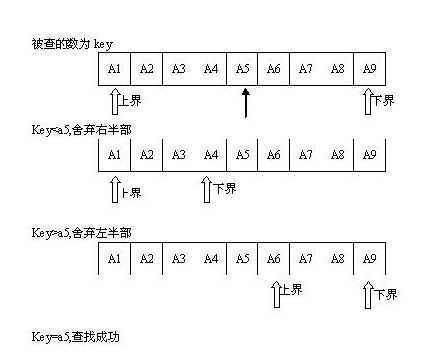int Binary_Search(int key,int low,int high)//折半查找
{
int mid;
if(low == high)
{
if(data[low] == key)
return low;
else
return -1;
}
else
{
mid = (low + high) / 2;
if(mid == low)
mid++;
if(key < data[mid])
return Binary_Search(key, low, mid - 1);
else
return Binary_Search(key, mid, high);
}
}

3.1.3斐波那契查找的前提是待查找的查找表必须顺序存储并且有序

1）相等,mid位置的元素即为所求
2）> ,low=mid+1;
3) < ,high=mid-1;

1）相等，mid位置的元素即为所求
2）> ,low=mid+1,k-=2;

3)< ,high=mid-1,k-=1;

void fibonacci(int *f)//斐波那契数
{
f = 1;
f = 1;
for(int i = 2;i < 20;++i)
f[i] = f[i - 2] + f[i - 1];
}
int fibonacci_search(int *a,int key,int n)//斐波那契查找
{
int low = 0,high = n - 1;
int mid = 0;
int k = 0;
int F;
fibonacci(F);
while(n > F[k] - 1) //计算出n在斐波那契中的数列
++k;
for(int i = n;i < F[k] - 1;++i) //把数组补全
a[i] = a[high];
while(low <= high)
{
mid = low + F[k-1] - 1; //根据斐波那契数列进行黄金分割
if(a[mid] > key)
{
high = mid - 1;
k = k - 1;
}
else if(a[mid] < key)
{
low = mid + 1;
k = k - 2;
}
else{
if(mid <= high) //如果为真则找到相应的位置
return mid;
else
return -1;
}
}
return -1;
}

1）当key=a[mid]时，查找成功；
2）当key<a[mid]时，新的查找范围是第low个到第mid-1个，此时范围个数为F[k-1] - 1个，即数组左边的长度，所以要在[low, F[k - 1] - 1]范围内查找；
3）当key>a[mid]时，新的查找范围是第mid+1个到第high个，此时范围个数为F[k-2] - 1个，即数组右边的长度，所以要在[F[k - 2] - 1]范围内查找。

3.1.4二叉排序树简介

1、若它的左子树不空，则左子树上所有节点的值均小于它的根节点的值；
2、若它的右子树不空，则右子树上所有节点的值均大于它的根节点的值；
3、它的左右子树也分别为二叉排序树。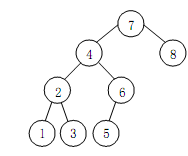3.2二叉排序树相关操作
3.2.1二叉排序树的操作介绍

1、若待删节点p为叶子节点，则删除p后，并不会破坏整棵树的结构，因此只需令p=NULL即可。
2、若待删节点p只有左子树或右子树，则只需将左子树或右子树重接到p的父节点上即可，即执行如下操作：p=p->lchild或p=p->rchild。
3、若待删节点p既有左子树又有右子树，显然就不如上面两种情况那么简单了。我们要使节点p被删除后，二叉排序树的结构不变，就需要对它的子树做一些操作，而且只需操作一个子树即可，操作左子树和操作右子树的思路相似。

typedef struct BSTNode
{
KeyType key;     //数据域
BSTNode *lchild;
BSTNode *rchild;
}


3.2.2二叉排序树的插入操作
(1)如果二叉排序树T为空，则创建一个关键字为k的结点，将其作为根结点。
(2)否则将k和根结点的关键字进行比较，如果相等则返回，如果k小于根结点的关键字则插入根结点的左子树中，否则插入根结点的右子树中。

   int InsertBST(BSTNode *p, KeyType k)
{
if(p==NULL)
{
p=(BSTNode*)malloc(sizeof(BSTNode));
p->key=k;
p->lchild=p->rchild=NULL;
return 1;
}
else if(k==p->key)
return 0;
else if(k<p->key)
return InsertBST(p->lchild, k);
else
return InsertBST(p->rchild, k);
}


{
BSTNode *bt=NULL;
int i=0;
while(i<n)
{
InsertBST(bt, A[i]);
i++;
}
return bt;
}输入{50,16,56,52,8}生成二叉排序树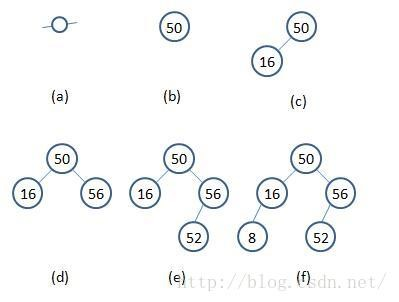3.2.3二叉排序树的查找操作

BSTree SearchBST(BSTree t, int k)
{
if(tnull || kt->key)
return t;
else if(kkey)
return SearchBST(t->lchild, k);
else
return SearchBST(t->rchild, k);
}

BSTree SearchBST2(BSTree t, int k)
{
BSTree p=t;
while(p!=null && p->key!=k)
{
if(kkey)
p=p->lchild;
else
p=p->rchild;
}
return p;
}

  ![这里写图片描述](https://img-blog.csdn.net/20180710145514842?watermark/2/text/aHR0cHM6Ly9ibG9nLmNzZG4ubmV0L3doajcwNzIxNjg1Mw==/font/5a6L5L2T/fontsize/400/fill/I0JBQkFCMA==/dissolve/70)


3.2.4二叉排序树的删除操作

1)查找待删除的结点

2)删除结点

(1)p为叶子结点。

p结点直接删除。
p为左子树：
f->lchild=NULL;
free§;
p为右子树:
f->rclild=NULl;

                          free(p);
操作示意图如下：![这里写图片描述](https://img-blog.csdn.net/20180710145547295?watermark/2/text/aHR0cHM6Ly9ibG9nLmNzZG4ubmV0L3doajcwNzIxNjg1Mw==/font/5a6L5L2T/fontsize/400/fill/I0JBQkFCMA==/dissolve/70)

(2)

*p只有左子树，或只有右子树。
对于这种情况，可以直接将*p的左子树或右子树与其双亲结点*f相连，然后删除*p。
p为f的左孩子，p的左子树非空：
f->lchild=p->lchild;
free(p);
p为f的左孩子，p的右子树非空：
f->lchild=p->rchild;
free(p);
p为f的右孩子，p的左子树非空：
f->rchild=p->lchild;
free(p);
p为f的右孩子，p的右子树非空：
f->rchild=p->rchild;

free(p);

    操作示意图如下：
![这里写图片描述](https://img-blog.csdn.net/20180710145636221?watermark/2/text/aHR0cHM6Ly9ibG9nLmNzZG4ubmV0L3doajcwNzIxNjg1Mw==/font/5a6L5L2T/fontsize/400/fill/I0JBQkFCMA==/dissolve/70)
(3)*p有左右子树。


f->lchild=p->lchild;
s->rchild=p->rchild;

                  free(p);
操作示意图如下：
![这里写图片描述](https://img-blog.csdn.net/20180710145704781?watermark/2/text/aHR0cHM6Ly9ibG9nLmNzZG4ubmV0L3doajcwNzIxNjg1Mw==/font/5a6L5L2T/fontsize/400/fill/I0JBQkFCMA==/dissolve/70)


1.对α的左儿子的左子树进行一次插入
2.对α的左儿子的右子树进行一次插入
3.对α的右儿子的左子树进行一次插入
4.对α的右儿子的右子树进行一次插入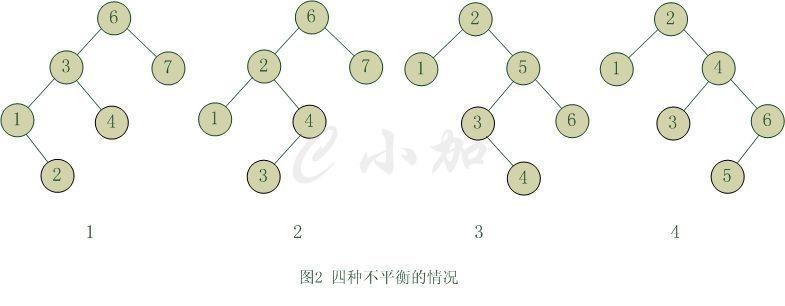①　单旋转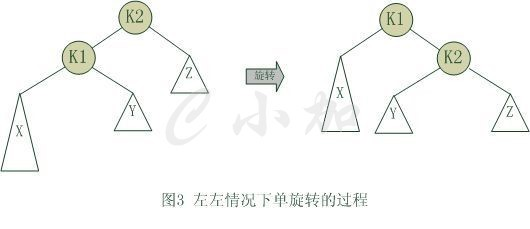②　双旋转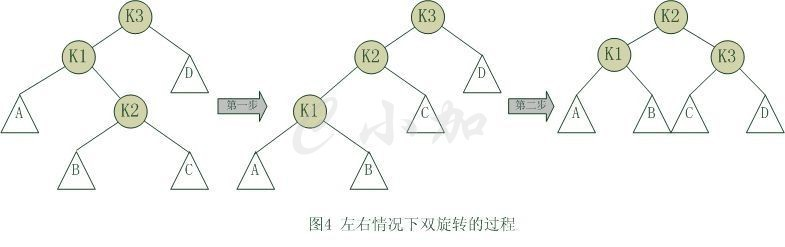AVL树的删除操作：

①　.要删除的节点是当前根节点T。

(1)、左子树高度大于右子树高度，将左子树中最大的那个元素赋给当前根节点，然后删除左子树中元素值最大的那个节点。
(2)、左子树高度小于右子树高度，将右子树中最小的那个元素赋给当前根节点，然后删除右子树中元素值最小的那个节点。

②　、要删除的节点元素值小于当前根节点T值，在左子树中进行删除。

③　、要删除的节点元素值大于当前根节点T值，在右子树中进行删除。
3.3功能模块设计

（1）直接定址法

（2）数字分析法

（3）平方取中法

（4）除留余数法

（5）随机数法

（1）开放定址法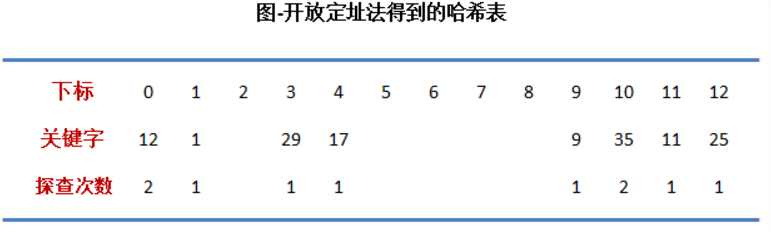a. 12 % 13 结果是12，而它的前面有个 25 ，25 % 13 也是12，存在冲突。

b. 35 % 13 结果是 9，而它的前面有个 9，9 % 13也是 9，存在冲突。

2）拉链法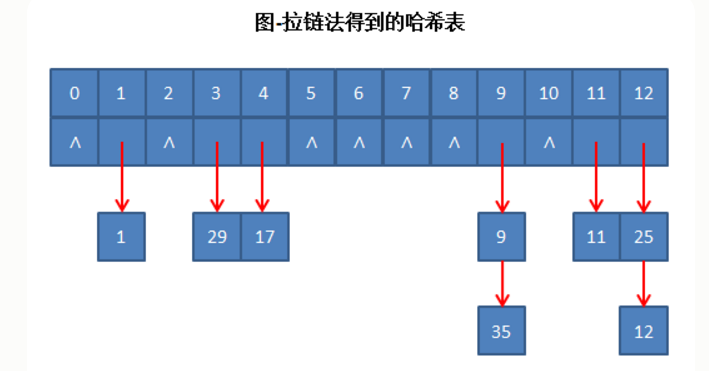1、线性探查

Hash(37) = 4 Hash(25) = 3 Hash(14) = 3 Hash(36) = 3 Hash(49) = 5 Hash(68) = 2 Hash(57) = 2 Hash(11) = 0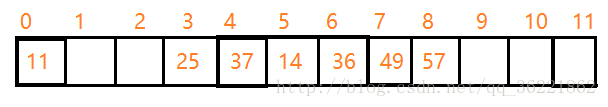α是散列表装满程度的标志因子。由于表长是定值，α与“填入表中的元素个数”成正比，所以，α越大，填入表中的元素较多，产生冲突的可能性就越大；α越小，填入表中的元素较少，产生冲突的可能性就越小

2、二次探查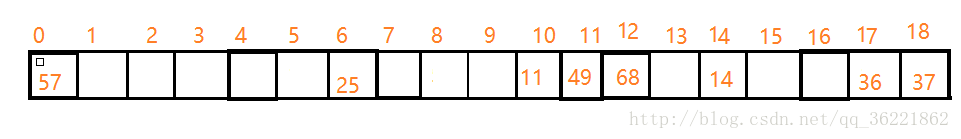typedef struct{  //哈希表线性探测再散列数据类型定义
int key;          //关键字
int si;       //插入成功时的次数
}HashTable1;

typedef struct Ha{   //哈希表链地址法数据类型定义
int elem;           //数据项
struct Ha *next2;   //指向下一个结点的指针
}HashTable2;

typedef struct{  //哈希表二次探测再散列法数据类型定义
int elem[HashSize];     //表中储存数据元素的数组
int count;              //表中储存数据元素的个数
int size;              //哈希表的尺寸
}HashTable3;


void CreateHashTable1(HashTable1 *H,int *a,int num) //哈希表线性探测再散列(除留余数法)
{
int i,d,cnt;
for(i=0; i<HashSize; i++)     //给新哈希表初始化数据
{
H[i].key=0;         H[i].si=0;
}
for(i=0; i<num; i++)
{
cnt=1;
d=a[i]%HashSize;     //构造哈希函数
if(H[d].key==0)      //无冲突时，直接插入数据
{
H[d].key=a[i];
H[d].si=cnt;
}
else{               //有冲突时，进行线性探测法解决冲突再插入
do{
d=(d+1)%HashSize;
cnt++;
}
while(H[d].key!=0);
H[d].key=a[i];
H[d].si=cnt;
}
}
printf("\n线性再探测哈希表已建成!\n");
}

void CreateHashTable2(HashTable2 *H,int *a,int num)
{
int key,i;
HashTable2 *q,*qq;
q=NULL;
for (i=0; i<HashSize; i++)              //对哈希表进行初始化
{
H[i].elem = 0;
H[i].next2=NULL;
}
for (i=0; i<num; i++)
{
key=a[i]%HashSize;
if(H[key].elem==0)
H[key].elem=a[i];
else
{
if(q!=NULL)     //若该位置已有数据
{
qq=q;
q=q+1;
q->elem=a[i];                //添加到已存在的结点后面
q->next2=qq->next2;
qq->next2=q;
}
else
{
q=(HashTable2*)malloc(sizeof(HashTable2));
if(!q)
{
printf("\n申请内存失败!请重新运行程序\n");
exit(1);
}
q->elem=a[i];                     //添加到首结点后面
q->next2=H[key].next2;
H[key].next2=q;
}
}
}
printf("\n链表探索哈希表已建成!\n");
}


void SearchHash1(HashTable1 *h,int data)
{
int d,i;
d=data%HashSize; //哈希函数
i=d;
if(h[d].key==data)     //一次查找成功
printf("\n数字%d的查找次数为：%d\n",h[d].key,h[d].si);
else
{
while(i<HashSize && h[d].key!=data )
{
d=(d+1)%HashSize;
i+=1;
}
if(i<HashSize)
printf("\n数字%d的查找次数为：%d.\n",h[d].key,h[d].si);
else
printf("\n没有找到您所输入的数\n");
}
}

int SearchHash2(HashTable2 *h,int data,int num)
{
int d,cnt=1;
HashTable2 *q;
d=data%HashSize;       //哈希函数
q=&h[d];
if(q->elem==0)         //该位置上没有数据元素
{
printf("\n没有找到您要查找的数\n");
return 0;
}
while(q!=NULL)
{
if(q->elem==data)
{
printf("\n数字%d的查找次数为：%d\n",data,cnt);
return 0;
}
else if(q->next2!=NULL)
{
q=q->next2;
cnt++;
}
else
{
printf("\n没有找到您要查找的数\n");
return 0;
}
}
return 0; 	printf("\n");}



4.运行与测试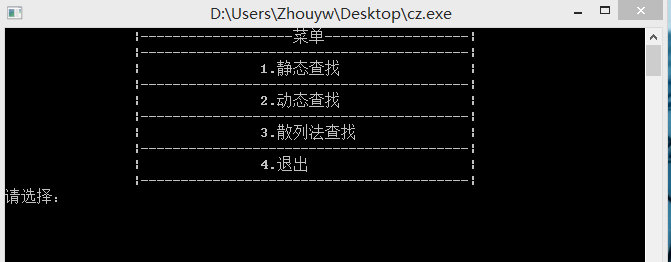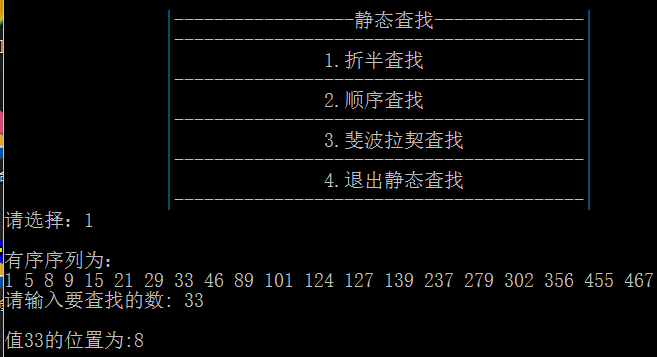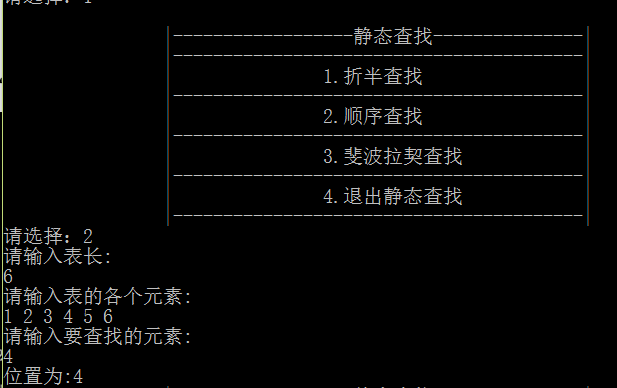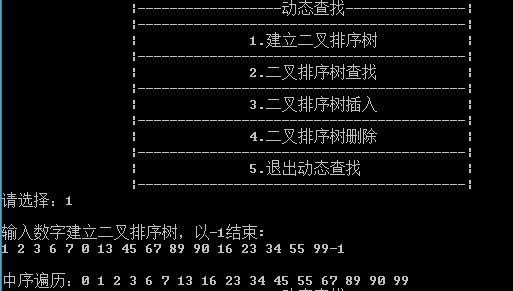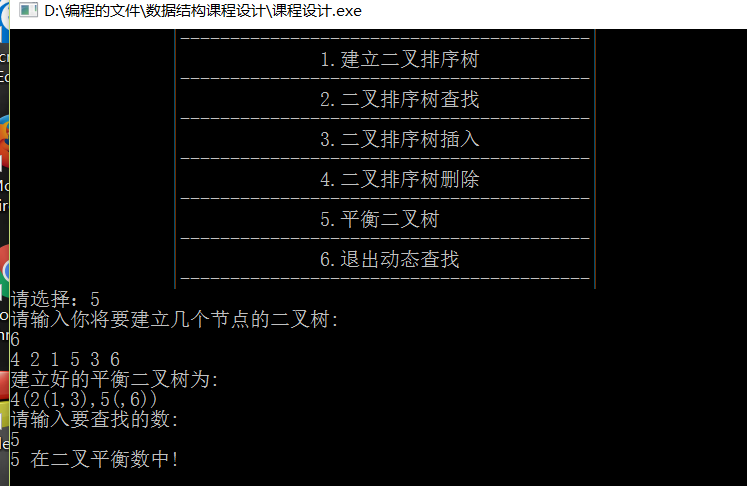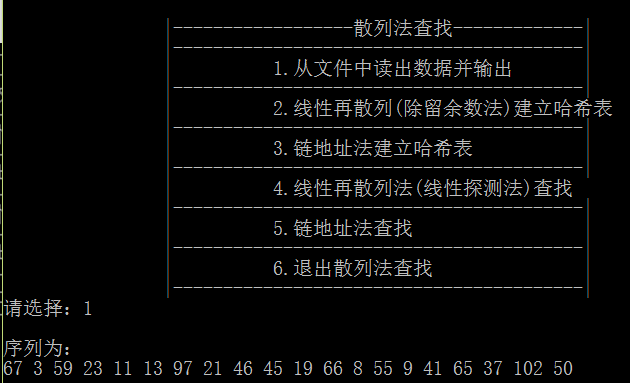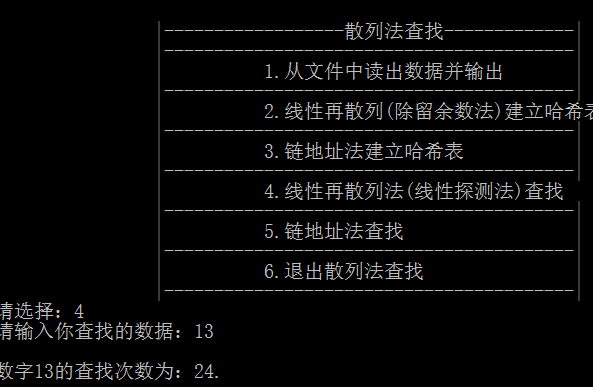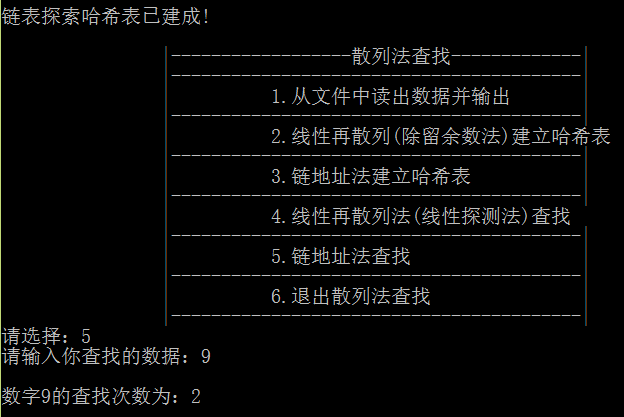5.总结

6.附录

#include<stdio.h>
#include<stdlib.h>
#include <fstream>
#include <iostream>
#include<cstdio>
#include<cstdlib>
using namespace std;
#define HashSize 50
#define MAX 100
#define LH 1
#define EH 0
#define RH -1
FILE *fp;
int data;
int data2;

//********************************************************************
int Binary_Search(int key,int low,int high)//折半查找
{
int mid;
if(low == high)
{
if(data[low] == key)
return low;
else
return -1;
}
else
{
mid = (low + high) / 2;
if(mid == low)
mid++;
if(key < data[mid])
return Binary_Search(key, low, mid - 1);
else
return Binary_Search(key, mid, high);
}
}

void zheban()
{
int locate;
int key;
if((fp=fopen("D:\\编程的文件\\查找算法性能比较\\折半查找.txt","r"))==NULL)
{
printf("cannot open file\n");
}
for(int i=0;i<20;i++)
fscanf(fp,"%d",&data[i]);
fclose(fp);
printf("\n有序序列为：\n");
for(int i=0;i<20;i++)
printf("%d ",data[i]);
printf("\n请输入要查找的数: ");
scanf("%d", &key);
if(key != -1)
{
locate = Binary_Search(key,0,19);
if(locate != -1)
{
printf("\n值%d的位置为:%d\n\n", key, locate+1);
}
else
{
printf("没有找到%d\n", key);
}
}
else
exit(1);
cout<<endl;
}
//************************************************************************
typedef struct
{
int key;   //关键字
int data;    //信息域
}Record;                                  //用给定的关键字值与表中其他元素的关键字值比较
typedef struct
{
Record r[MAX+1];
int length;
}SqTable;
int search(SqTable s,int k)
{
int i;
s.r.key=k;
i=s.length;      //自几用的逆序
while(s.r[i].key!=k)
i--;
return i;
}
void sunxu()
{
SqTable s;
int wz,i,len,x,y;
printf("请输入表长:\n");
scanf("%d",&len);
s.length=len;
printf("请输入表的各个元素:\n");
for(i=1;i<=len;i++)
{
scanf("%d",&x);
s.r[i].key=x;
}
printf("请输入要查找的元素:\n");
scanf("%d",&y);
wz=search(s,y);
if(wz==0)
{
printf("不存在:\n");
}
else
printf("位置为:%d",wz);
}
//************************************************************************
void fibonacci(int *f)//斐波那契数
{
f = 1;
f = 1;
for(int i = 2;i < 20;++i)
f[i] = f[i - 2] + f[i - 1];
}
int fibonacci_search(int *a,int key,int n)//斐波那契查找
{
int low = 0,high = n - 1;
int mid = 0;
int k = 0;
int F;
fibonacci(F);
while(n > F[k] - 1) //计算出n在斐波那契中的数列
++k;
for(int i = n;i < F[k] - 1;++i) //把数组补全
a[i] = a[high];
while(low <= high)
{
mid = low + F[k-1] - 1;  //根据斐波那契数列进行黄金分割
if(a[mid] > key)
{
high = mid - 1;
k = k - 1;
}
else if(a[mid] < key)
{
low = mid + 1;
k = k - 2;
}
else{
if(mid <= high) //如果为真则找到相应的位置
return mid;
else
return -1;
}
}
return -1;
}
int Fibonacci()
{
if((fp=fopen("D:\\编程的文件\\查找算法性能比较\\斐波那契.txt","r"))==NULL)
{
printf("cannot open file\n");
}
for(int i=0;i<20;i++)
fscanf(fp,"%d",&data[i]);
fclose(fp);
printf("\n有序序列为：\n");
for(int i=0;i<20;i++)
printf("%d ",data[i]);
printf("\n");
int k;
printf("\n请输入要查找的数:\n");
scanf("%d",&k);
int pos = fibonacci_search(data,k,20);
if(pos != -1)
printf("\n值%d的位置为：%d\n\n", k, pos+1);
else
printf("没有找到%d\n", k);
return 0;
}
//**************************************************************************
struct node//二叉排序树
{
int data;
};

struct node *tree;

void inordertraverse(struct node *tree)
{
if(tree)
{
cout<<tree->data<<" ";
}
}

struct node *find(struct node *tree, int x)  //二叉排序树的查找算法
{
int f;
struct node *p,*q;
p=tree;
f=0;
q=( struct node *)malloc(sizeof (struct node));
while((!f)&&(p!=NULL))
{
if(p->data==x)
f=1;
else
if(x<p->data)
else
}
if(f)
q=p;
else
q->data=NULL;
return(q);
}

struct node *findparents(struct node *tree, int x)  //二叉排序树双亲节点的查找算法
{
int f;
struct node *p,*q,*r;
p=tree;
f=0;
q=( struct node *)malloc(sizeof (struct node));
r=( struct node *)malloc(sizeof (struct node));
r=NULL;
while((!f)&&(p!=NULL))
{
if(p->data==x)
f=1;
else
{
r=p;
if(x<p->data)
{
}
else
{
}
}
}
if(f)
q=p;
else
q->data=NULL;
return(r);
}
struct node * insertree(struct node *tree,  int x )  //插入
{
struct node *p,*q;
if(tree==NULL)
{
p= (struct node *)malloc(sizeof(struct node));
p->data=x;
tree=p;
}
else
{  p=tree;
while(p!=NULL)
{
q=p;
if (x<p->data)
else
}
p=(struct node *)malloc(sizeof(struct node));
p->data=x;
if (x<q->data)
else
}
return tree;
}

void detree(struct node *t, struct node *f, struct node *p)  //删除
{
struct node *q,*s;
int boo;
boo=0;
{
{
if(p==t)
else
{
boo=1;
}
}
else
{
if(p==t)
else
{
boo=1;
}
}
}
else
{
q=p;
{
q=s;
}
if (q!=p)
{
}
if(p==t)
t=s;
else
boo=1;
}
if (boo==1)
{
else
}
free(p);
}
//**************************************************************************
typedef struct BTNode
{
int data;
int BF;//平衡因子（balance factor）
struct BTNode *lchild,*rchild;
}BTNode,*BTree;
void R_Rotate(BTree *p)//以p为根节点的二叉排序树进行右旋转
{
BTree L;
L=(*p)->lchild;
(*p)->lchild=L->rchild;
L->rchild=(*p);
*p=L;//p指向新的根节点
}
void L_Rotate(BTree *p)//以p为根节点的二叉排序树进行左旋转
{
BTree R;
R=(*p)->rchild;
(*p)->rchild=R->lchild;
R->lchild=(*p);
*p=R;
}
void LeftBalance(BTree *T)
{
BTree L,Lr;
L=(*T)->lchild;
switch(L->BF)
{
//检查T的左子树平衡度，并作相应的平衡处理
case LH://新节点插入在T的左孩子的左子树上，做单右旋处理
(*T)->BF=L->BF=EH;
R_Rotate(T);
break;
case RH://新插入节点在T的左孩子的右子树上，做双旋处理
Lr=L->rchild;
switch(Lr->BF)
{
case LH:
(*T)->BF=RH;
L->BF=EH;
break;
case EH:
(*T)->BF=L->BF=EH;
break;
case RH:
(*T)->BF=EH;
L->BF=LH;
break;
}
Lr->BF=EH;
L_Rotate(&(*T)->lchild);
R_Rotate(T);
}
}
void RightBalance(BTree *T)
{
BTree R,Rl;
R=(*T)->rchild;
switch(R->BF)
{
case RH://新节点插在T的右孩子的右子树上，要做单左旋处理
(*T)->BF=R->BF=EH;
L_Rotate(T);
break;
case LH://新节点插在T的右孩子的左子树上，要做双旋处理
Rl=R->lchild;
switch(Rl->BF)
{
case LH:
(*T)->BF=EH;
R->BF=RH;
break;
case EH:
(*T)->BF=R->BF=EH;
break;
case RH:
(*T)->BF=LH;
R->BF=EH;
break;
}
Rl->BF=EH;
R_Rotate(&(*T)->rchild);
L_Rotate(T);
}
}
bool InsertAVL(BTree *T,int e,bool *taller)//变量taller反应T长高与否
{
if(!*T)
{
*T=(BTree)malloc(sizeof(BTNode));
(*T)->data=e;
(*T)->lchild=(*T)->rchild=NULL;
(*T)->BF=EH;
*taller=true;
}
else
{
if(e==(*T)->data)//不插入
{
*taller=false;
return false;
}
if(e<(*T)->data)
{
if(!InsertAVL(&(*T)->lchild,e,taller))//未插入
return false;
if(*taller)//以插入左子树，且左子树变高
{
switch((*T)->BF)
{
case LH://原本左子树比右子树高，需要做左平衡处理
LeftBalance(T);
*taller=false;
break;
case EH://原本左右子树等高，现因左子树增高而树增高
(*T)->BF=LH;
*taller=true;
break;
case RH://原本右子树比左子树高，现在左右子树等高
(*T)->BF=EH;
*taller=false;
break;
}
}
}
else
{
//应在T的右子树中搜寻
if(!InsertAVL(&(*T)->rchild,e,taller))
return false;
if(*taller)//插入右子树，且右子树长高
{
switch((*T)->BF)
{
case LH://原本左子树比右子树高，现在左右子树等高
(*T)->BF=EH;
*taller=false;
break;
case EH://原本左右子树等高，现在右子树变高
(*T)->BF=RH;
*taller=true;
break;
case RH://原本右子树比左子树高，现在需做右平衡处理
RightBalance(T);
*taller=false;
break;
}
}
}
}
return true;
}
bool Find(BTree T,int key)
{
if(!T)
return false;
else if(T->data==key)
return true;
else if(T->data<key)
return Find(T->rchild,key);
else
return Find(T->lchild,key);
}
void Output(BTree T)
{
if(T)
{
printf("%d",T->data);
if(T->lchild||T->rchild)
{
printf("(");
Output(T->lchild);
printf(",");
Output(T->rchild);
printf(")");
}
}
}
void pingheng()
{
int i,n;
printf("请输入你将要建立几个节点的二叉树:\n");
scanf("%d",&n);
int A[n];
int a,b;
for(i=0;i<n;i++)
{
scanf("%d",&a);
A[i]=a;
}
BTree T=NULL;
bool taller;
for(i=0;i<sizeof(A)/sizeof(int);i++)
InsertAVL(&T,A[i],&taller);
printf("建立好的平衡二叉树为:\n");
Output(T);
printf("\n");
printf("请输入要查找的数:\n");
scanf("%d",&b);
if(Find(T,b))
printf("%d 在二叉平衡数中!\n",b);
else
printf("%d 不在二叉平衡数中!\n",b);
}

//********************************************************

//散列法
typedef struct{  //哈希表线性探测再散列数据类型定义
int key;          //关键字
int si;       //插入成功时的次数
}HashTable1;

typedef struct Ha{   //哈希表链地址法数据类型定义

int elem;           //数据项
struct Ha *next2;   //指向下一个结点的指针
}HashTable2;

typedef struct{  //哈希表二次探测再散列法数据类型定义
int elem[HashSize];     //表中储存数据元素的数组
int count;              //表中储存数据元素的个数
int size;              //哈希表的尺寸
}HashTable3;

void CreateHashTable1(HashTable1 *H,int *a,int num) //哈希表线性探测再散列(除留余数法)
{
int i,d,cnt;
for(i=0; i<HashSize; i++)     //给新哈希表初始化数据
{
H[i].key=0;         H[i].si=0;
}
for(i=0; i<num; i++)
{
cnt=1;
d=a[i]%HashSize;     //构造哈希函数
if(H[d].key==0)      //无冲突时，直接插入数据
{
H[d].key=a[i];
H[d].si=cnt;
}
else{               //有冲突时，进行线性探测法解决冲突再插入
do{
d=(d+1)%HashSize;
cnt++;
}
while(H[d].key!=0);
H[d].key=a[i];
H[d].si=cnt;
}
}
printf("\n线性再探测哈希表已建成!\n");
printf("散列表的位置:\n");
for(i=0;i<20;i++)
printf("%d  ",i);
printf("\n");
printf("关键字:\n");
for(i=0;i<20;i++)
printf("%d  ",H[i].key);
printf("\n");
}

void CreateHashTable2(HashTable2 *H,int *a,int num)
{
int key,i;
HashTable2 *q,*qq;
q=NULL;
for (i=0; i<HashSize; i++)              //对哈希表进行初始化
{
H[i].elem = 0;
H[i].next2=NULL;
}
for (i=0; i<num; i++)
{
key=a[i]%HashSize;
if(H[key].elem==0)
H[key].elem=a[i];
else
{
if(q!=NULL)     //若该位置已有数据
{
qq=q;
q=q+1;
q->elem=a[i];                //添加到已存在的结点后面
q->next2=qq->next2;
qq->next2=q;
}
else
{
q=(HashTable2*)malloc(sizeof(HashTable2));
if(!q)
{
printf("\n申请内存失败!请重新运行程序\n");
exit(1);
}
q->elem=a[i];                     //添加到首结点后面
q->next2=H[key].next2;
H[key].next2=q;
}
}
}
printf("\n链表探索哈希表已建成!\n");
}

void SearchHash1(HashTable1 *h,int data)
{
int d,i;
d=data%HashSize; //哈希函数
i=d;
if(h[d].key==data)     //一次查找成功
printf("\n数字%d的查找次数为：%d\n",h[d].key,h[d].si);
else
{
while(i<HashSize && h[d].key!=data )
{
d=(d+1)%HashSize;
i+=1;
}
if(i<HashSize)
printf("\n数字%d的查找次数为：%d.\n",h[d].key,h[d].si);
else
printf("\n没有找到您所输入的数\n");
}
}

int SearchHash2(HashTable2 *h,int data,int num)
{
int d,cnt=1;
HashTable2 *q;
d=data%HashSize;       //哈希函数
q=&h[d];
if(q->elem==0)         //该位置上没有数据元素
{
printf("\n没有找到您要查找的数\n");
return 0;
}
while(q!=NULL)
{
if(q->elem==data)
{
printf("\n数字%d的查找次数为：%d\n",data,cnt);
return 0;
}
else if(q->next2!=NULL)
{
q=q->next2;
cnt++;
}
else
{
printf("\n没有找到您要查找的数\n");
return 0;
}
}
return 0;
printf("\n");
}

void GetIn()
{
if((fp=fopen("D:\\编程的文件\\查找算法性能比较\\散列法.txt","r"))==NULL)   //
{
printf("cannot open file\n");
}
for(int i=0;i<20;i++)
fscanf(fp,"%d",&data2[i]);
fclose(fp);
printf("\n序列为：\n");
for(int i=0;i<20;i++)
printf("%d ",data2[i]);
printf("\n");
}

{
int m;
cout<<endl;
cout<<"\t\t|------------------静态查找---------------|"<<endl;
cout<<"\t\t|-----------------------------------------|"<<endl;
cout<<"\t\t|\t\t1.折半查找\t\t  |"<<endl;
cout<<"\t\t|-----------------------------------------|"<<endl;
cout<<"\t\t|\t\t2.顺序查找\t\t  |"<<endl;
cout<<"\t\t|-----------------------------------------|"<<endl;
cout<<"\t\t|\t\t3.斐波拉契查找\t\t  |"<<endl;
cout<<"\t\t|-----------------------------------------|"<<endl;
cout<<"\t\t|\t\t4.退出静态查找\t\t  |"<<endl;
cout<<"\t\t|-----------------------------------------|"<<endl;
cout<<"请选择：";
cin>>m;
while(m<1||m>4)
{
cout<<"输入有误，请重新输入！";
cin>>m;
}
switch(m)
{
case 1:
zheban();
break;
case 2:
sunxu();
break;
case 3:
Fibonacci();
break;
case 4:
cout<<"退出静态查找！"<<endl;
system("cls");
}
}

{
int m;
cout<<endl;
cout<<"\t\t|------------------动态查找---------------|"<<endl;
cout<<"\t\t|-----------------------------------------|"<<endl;
cout<<"\t\t|\t       1.建立二叉排序树\t\t  |"<<endl;
cout<<"\t\t|-----------------------------------------|"<<endl;
cout<<"\t\t|\t       2.二叉排序树查找\t\t  |"<<endl;
cout<<"\t\t|-----------------------------------------|"<<endl;
cout<<"\t\t|\t       3.二叉排序树插入\t\t  |"<<endl;
cout<<"\t\t|-----------------------------------------|"<<endl;
cout<<"\t\t|\t       4.二叉排序树删除\t\t  |"<<endl;
cout<<"\t\t|-----------------------------------------|"<<endl;
cout<<"\t\t|\t       5.平衡二叉树   \t\t  |"<<endl;
cout<<"\t\t|-----------------------------------------|"<<endl;
cout<<"\t\t|\t       6.退出动态查找\t\t  |"<<endl;
cout<<"\t\t|-----------------------------------------|"<<endl;
cout<<"请选择：";
cin>>m;
while(m<1||m>6)
{
cout<<"\n输入有误，请重新输入:\n";
cin>>m;
}
switch(m)
{
case 1:
{
tree=(struct node*)malloc(sizeof(struct node));
tree =NULL;
cout<<"\n输入数字建立二叉排序树，以-1结束:"<<endl;
while(1)
{
int y;
cin>>y;
if(-1==y)
break;
tree=insertree(tree,y);
}
cout<<"\n中序遍历：";
inordertraverse(tree);
cout<<endl;
case 2 :                          //查找
{
int x;
cout<<"\n请输入您要查找的数："<<endl;
cin>>x;
if(find(tree, x)->data==x)
cout<<"\n已找到您要查找的数！"<<find(tree,x)->data<<endl;
else
cout<<"\n未找到您要查找的数！"<<endl;
case 3 :                         //插入
{
int x;
cout<<"\n请输入您要插入的数"<<endl;
cin>>x;
insertree(tree, x );
cout<<"\n中序遍历：";
inordertraverse(tree) ;
cout<<endl;
case 4:                            //删除
{
cout<<"\n请输入您要删除的数据"<<endl;
struct node *f,*p;
int x;
cin>>x;
p=find(tree, x);
f=findparents(tree, x);
detree(tree, f, p);
cout<<"\n中序遍历：";
inordertraverse(tree) ;
cout<<endl;
case 5:
{
pingheng();
case 6:
cout<<"退出动态查找！"<<endl;
}
system("cls");
}

{   int m,d,i;
HashTable1 hash1[HashSize];
HashTable2 hash2[HashSize];
HashTable3 * ha;                    //定义三种类型的哈希表
ha=(HashTable3 *)malloc(sizeof(HashTable3));
for(i=0; i<HashSize; i++)//哈希表的初始化
{
ha->elem[i]=0;
ha->count=0;
}
ha->size=HashSize;
while(1)
{
cout<<endl;
cout<<"\t\t|------------------散列法查找-------------|"<<endl;
cout<<"\t\t|-----------------------------------------|"<<endl;
cout<<"\t\t|\t   1.从文件中读出数据并输出\t  |"<<endl;
cout<<"\t\t|-----------------------------------------|"<<endl;
cout<<"\t\t|\t   2.线性再散列(除留余数法)建立哈希表\t  |"<<endl;
cout<<"\t\t|-----------------------------------------|"<<endl;
cout<<"\t\t|\t   3.链地址法建立哈希表\t\t  |"<<endl;
cout<<"\t\t|-----------------------------------------|"<<endl;
cout<<"\t\t|\t   4.线性再散列法(线性探测法)查找\t\t  |"<<endl;
cout<<"\t\t|-----------------------------------------|"<<endl;
cout<<"\t\t|\t   5.链地址法查找\t\t  |"<<endl;
cout<<"\t\t|-----------------------------------------|"<<endl;
cout<<"\t\t|\t   6.退出散列法查找\t\t  |"<<endl;
cout<<"\t\t|-----------------------------------------|"<<endl;
cout<<"请选择：";
cin>>m;
while(m<1||m>6)
{
cout<<"输入有误，请重新输入:";
cin>>m;
}
switch(m)
{
case 1:
GetIn();
break;
case 2:
CreateHashTable1(hash1,data2,20);
break;
case 3:
CreateHashTable2(hash2,data2,20);
break;
case 4:
printf("请输入你查找的数据：");
scanf("%d",&d);
SearchHash1(hash1,d);
break;
case 5:
printf("请输入你查找的数据：");
cin>>d;
SearchHash2(hash2,d,20);
break;
case 6:
system("cls");
}
}

}
{
int m;
cout<<"\t\t|-------------------菜单------------------|"<<endl;
cout<<"\t\t|-----------------------------------------|"<<endl;
cout<<"\t\t|\t\t1.静态查找\t\t  |"<<endl;
cout<<"\t\t|-----------------------------------------|"<<endl;
cout<<"\t\t|\t\t2.动态查找\t\t  |"<<endl;
cout<<"\t\t|-----------------------------------------|"<<endl;
cout<<"\t\t|\t\t3.散列法查找\t\t  |"<<endl;
cout<<"\t\t|-----------------------------------------|"<<endl;
cout<<"\t\t|\t\t4.退出\t\t\t  |"<<endl;
cout<<"\t\t|-----------------------------------------|"<<endl;
cout<<"请选择：";
cin>>m;
while(m<1||m>4) {cout<<"输入有误，请重新输入:";cin>>m;}
switch(m)
{
case 1:
break;
case 2:
break;
case 3:
break;
case 4:
cout<<"退出程序！";
exit(0);
}
}

int main()
{
return 0;
}



## 这里是完整的设计报告和和代码